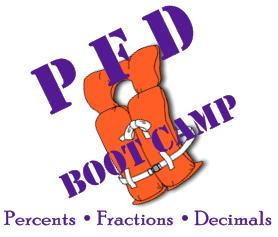Fractions
What are they?## This module is an introduction to the basics of fractions.            Module #1

Understanding fractions is a useful skill because

• Fractions are common in everyday life, from measuring in the wood shop to measuring sugar in the kitchen to finding a shooting percentage.
• Fractions come up in many settings and we often need to simplify them and put them into decimal or percent form. For example, if a baseball player has 56 hits in 160 at-bats, 56/160 = 7/20 = .350.
• It is important to understand how fractions work.
• Fractions are useful numbers for solving many problems.

6-question Pre-Quiz (answers may be obtained from Dr. Olsen)

All exercises in this module should be done without using a calculator. To fully understand how fractions work you need to be able to do the operations by hand (on paper or mentally). Once the concepts and procedures are mastered, there will be times that calculator use is appropriate.## Objectives

In this module you will learn

1. The meaning of a fraction.
2. How to put a fraction into lowest terms.
3. How to put a fraction in higher terms.
4. The fraction division relationship.
5. How to put a mixed number to improper fraction.# Background Knowledge

## Terms

• Numerator is the top number of the fraction.
• Denominator is the bottom number of the fraction.
• In division, the number you dividing by is the divisor. The answer is the quotient. (The beginning number is call the "dividend," but this term isn't used as often as the other terms.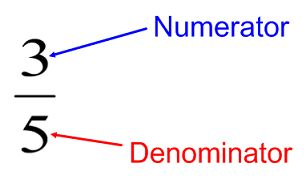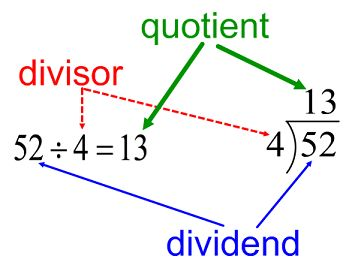Fractions can be written with a horizontal line or the "slash," which is common with computers. For example, three-fifths,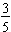, can be written 3/5.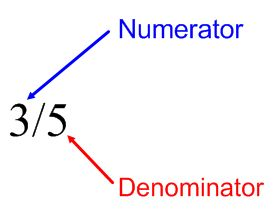Click here to go to my fractions page which has a Review Sheet.## So, Just What is a Fraction? - The meaning of a fraction.

A fraction is made up of a numerator (top number) and a denominator (bottom number).

• The denominator (bottom number) tells how many equal parts make up one whole.
• The numerator (top number) tells how many of these parts you actually have.

This is explained in the following video, which uses the Fraction Models from NCTM## Check for Understanding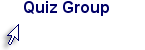## How to put a fraction into lowest terms and into higher terms.

Watch the following video.## Check for Understanding## The fraction division relationship.

Fractions can be thought of as a certain number of parts of a whole (and the number of parts could be greater than the number of parts to make up the whole). Fractions also represent division. Watch the following short video.## How to Convert a Mixed Number to an Improper Fraction

Watch the following video.## Try These

Here is a video on how to use www.aaamath.com.

## Practice Converting a Mixed Number to an Improper Fraction

Got www.aaamath.com (Fractions|Converting from Mixed Numbers). The practice area looks like the following: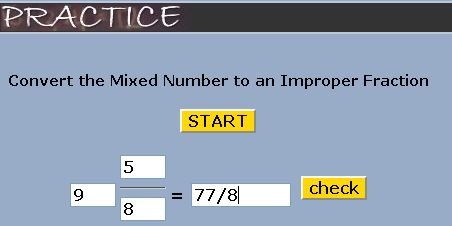## Post-Quiz

6-question Post-Quiz (answers may be obtained from Dr. Olsen)## Does This All Relate and Make Sense?

Fractions are found in everyday life and in math frequently. This module explained the meaning of fractions and how to put fractions into lowest terms and into higher terms. This will be especially important when we add fractions (in a future module). We also showed that fractions also represent division. This will be important for converting fractions to decimals (in a future module).

 This is module #1 in the PFD Boot Camp (overview). The PFD Boot Camp is on percents, fractions, and decimals. This module is an introction to fractions. The objectives covered in this module are found on page 2. The plan is to develop nine modules. The modules are under construction. If you want to brush up and practice the basic facts (+ - × ÷) click Basic Training. © Jim Olsen